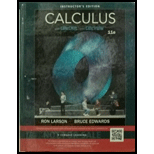Chapter 8.1, Problem 72E### Calculus (MindTap Course List)

11th Edition
Ron Larson + 1 other
ISBN: 9781337275347

#### Solutions

Chapter
Section### Calculus (MindTap Course List)

11th Edition
Ron Larson + 1 other
ISBN: 9781337275347
Textbook Problem

# Evaluating a Definite Integral In Exercises 57-72, evaluate the definite integral. Use a graphing utility to verify your result. ∫ 0 t 7 x 2 + 2 x ( x + 1 )   d x

To determine

To calculate: The definite integral and verify result by using the graphing utility.

Explanation

Given: 017x2+2x(x+1)dx

Calculation:

Consider the integral,

017x2+2x(x+1)dx

Substitute,

u=x2+2xdu=(2x+2)dx12du=(x+1)dx

Therefore,

017x2+2x(x+1)dx=12017udu

Apply the integration formula,

audu=aulna+c

Hence,

017x2+2x(x<

### Still sussing out bartleby?

Check out a sample textbook solution.

See a sample solution

#### The Solution to Your Study Problems

Bartleby provides explanations to thousands of textbook problems written by our experts, many with advanced degrees!

Get Started

## Additional Math Solutions

#### Find more solutions based on key concepts